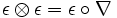# Bialgebra

## Definition

A bialgebra over a field$K$ is defined as a set equipped with the structure of a unital associative algebra over$K$ as well as a coalgebra over$K$ satisfying certain compatibility conditions.

### Notation

• Let$B$ be the set and$K$ be the underlying field
• Let$\nabla$ denote the multiplication and$\eta$ the unit of$B$ (for its algebra structure)
• Let$\Delta$ denote the comultiplication and$\epsilon$ the counit of$B$.
• Let$\tau$ be the unique linear map from$B \otimes B$ to itself that sends each pure tensor$x \otimes y$ to$y \otimes x$.

### Compatibility conditions

The compatibility conditions are as follows:

• Compatibility between multiplication and comultiplication:$\Delta \circ \nabla = (\nabla \otimes \nabla) \circ (id \otimes \tau \otimes id) \circ (\Delta \otimes \Delta)$

• Compatibility between multiplication and counit:$\epsilon \otimes \epsilon = \epsilon \circ \nabla$ under the canonical identification of$K \otimes K$ with$K$.

• Compatibility between comultiplication and unit:$\Delta \circ \eta = \eta \otimes \eta$

• Compatibility between unit and counit:$\epsilon \circ \eta = id$